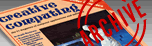`CREATIVE COMPUTING VOL. 11, NO. 4 / APRIL 1985 / PAGE 16`

 Statistical programs in Basic. (book reviews) Russ Lockwood. Designed with the student in mind, this book provides step-by-step Basic programming instructions for solving statistical problems on a computer. It assumes you already understand statistics, and presents a problem, the appropriate algorithm, a full listing of a program, and several example exercises. The "text" consists of REM statements within the programs, each explaining why a particular command is used. Topics include summation notation, data analysis, probability, probability distributions, estimation theory, hypothesis testing, regression and correlation, analysis of variance, and nonparametric tests. Schwartz, a mathematics professor, and Basso, a manager of information systems development, provide a no-nonsense approach to statistics problem solving. Review Grade: B